# Memory management in Extempore

It’s not really possible to explain Extempore’s memory allocation story without a detour into xtlang types, so we’ll cover some of that as well.

The two languages hosted by the Extempore compiler, xtlang and Scheme, have different approaches to allocating & managing memory. Both languages ultimately share the same memory—the stack and heap associated with the Extempore process—but via different mechanisms.

Broadly speaking, with Scheme code Extempore manages memory for you, while in xtlang you have to do it yourself. This is a common trade-off, and each has its advantages (in performance, programmer productivity, etc.) and disadvantages. So if you’re mostly going to be writing Scheme code (e.g. you’re making music using the built-in instruments) then you probably don’t need to read this (although understanding how things work under the hood is still sometimes helpful). To work effectively in xtlang, though, you’ll need to now a bit more about memory in Extempore.

## Automatic garbage collection in Scheme

Scheme objects (lists, closures, numbers, etc.) are automatically garbage collected by the Extempore run-time garbage collector (GC). This means that when new objects are created, memory is automatically allocated to store those objects, and as objects are destroyed or go out of scope (that is, there are no longer any references to them) the memory is automatically freed up for re-use.

Let’s do the most basic memory allocation imaginable: just binding a numerical value to a symbol.

``````(define a 5)

(println 'a '= a)  ;; prints a = 5
``````

The fact that we can use the symbol `a` and have it evaluate to `5` (as it should) means that the value (`5`) must be stored in memory somewhere. It doesn’t matter where in memory (what the address is), because we can always refer to the value using the symbol `a`. But it’s good to remember that the `define` form is allocating some memory, storing the value `5` in that memory, and binding a reference to the value in the symbol `a`.

We can redefine the symbol `a` to be some other Scheme object, say, a list.

``````(define a '(1 2 3))

(println 'a '= a)  ;; prints a = (1 2 3)
``````

The three-element list `(1 2 3)` takes up more memory than the number `5`. So `define` can’t just write the new value of `a` over the top of the old one. What it does (and in fact what re-defining things always does) is allocate some new memory to store the new value into, and change the variable `a` to point to that new value.

But what happens to the old value of `5` in memory? Well, it sits there untouched, at least for a while. But we can’t reach it—the only ‘handle’ we had to refer to it with was the symbol `a`, and that’s now bound to some other value instead. The value `5` in memory is ‘unreachable’. So there’s no point having it sitting around, taking up space.

That’s where the garbage collector comes in. Every now and then the garbage collector checks all the Scheme objects in the world, determines which of them are no longer reachable, and then frees up that memory to be used for other things. While I don’t recommend this harsh utilitarian approach to dealing with relatives who are down on their luck, it is good idea in a computer program. Memory is a finite resource, and the more efficiently we can get rid of memory that’s not being used the better.

Basically, having a GC means that when you’re writing Scheme code, you don’t have to worry about memory. The GC takes care of all the allocation/deallocation bookkeeping for you. The cost is that this bookkeeping requires CPU cycles—cycles which you could be using to do other cool things. Also, every now and then the GC has to briefly ‘stop the world’ (freeze the execution of all Scheme code) to do its job. This takes time, and introduces an element of uncertainty (non-determinism) to the execution of your code—you never know exactly when the GC is going to freeze things to do it’s job, and there’s a risk that it’ll happen at a really inconvenient time as far as your program is concerned (Murphy’s law and all that). This is particularly problematic in domains where timing is critical, such as real-time audio and video.

## Manual memory management in xtlang

Hang on a sec—isn’t working with real-time audio and video xtlang (and therefore Extempore’s) raison d’etre? Well, yes is is—the sluggishness (and non-determinism) of Impromptu’s Scheme interpreter was the spark which ignited the development of xtlang (as mentioned in philosophy). Again, this isn’t a knock on Scheme in general as slow—there are some very sprightly Scheme compilers, but Impromptu’s one was slow. The non-determinism was even more of a problem, because the last thing you want when you’re generating audio or video is a ‘stop the world’ GC pause, which will lead to clicks and pops in audio or dropped frames in video. Real-time systems and garbage collection are uneasy bedfellows.

So, xtlang requires manual memory management. In general, this means that when you want some memory you ask the compiler for it, it’s yours for a time and you can do whatever you want with it, and then you know when it’s going to be ‘given back’. It doesn’t necessarily mean that you have it forever (in fact in many cases the memory is quite short-lived), but it does mean that there are no surprises—you specify exactly how much memory you’ll get and you know it’s going to hang around for. This determinism an important benefit of manual memory management in xtlang—especially in a real-time systems context.

Zooming out for a second, a running program has access to and uses two main regions of memory: the stack and the heap. There’s lots of material on the web about the differences between these two a (here’s an explanation at stackoverflow), but I’ll give a quick summary here.

• The stack is for dealing with function arguments and local variables. Each function call ‘pushes’ some new data onto the stack, and when the function returns it ‘pops’ off any local variables and leaves its return value. The stack is therefore generally changing pretty quickly.
• The heap, on the other hand, is for longer-lived data. Buffers of audio, video, or any data which you want to have around for a while: these are the sort of things you’ll generally want to store on the heap.

I should also point out that the stack and heap aren’t actually different types of memory in the computer—they’re just different areas in the computer’s RAM. The difference is in the way the program uses the different regions. Each running process has its own stack(s) and heap, and they are just regions of memory given to the process by the OS.

So, that’s the stack and the heap, but there’s actually one other type of memory in Extempore: zone memory. A zone is a region of memory which can be easily deallocated all at once. So, if you have some data that you need to hang around longer than a function call (so a stack allocation is no good), but want to be able to conveniently deallocate all at once, then use a zone. There can be multiple zones in existence at once, and they don’t interfere (or have anything to do with) each other.

## The three flavours of memory in Extempore

So, in accordance with the three different memory ‘types’ (stack, heap, and zone) there are three memory allocation functions in xtlang: `salloc`, `halloc` and `zalloc`. They all return a pointer to some allocated memory, but they differ in where that memory is allocated from, and there are no prizes in guessing which function is paired with which type of memory :)

Also, `alloc` in xtlang is an alias for `zalloc`. So if you ever see an `alloc` in xtlang code it’s grabbing memory from a zone.

## Stack allocation with salloc

As I mentioned above, the stack is associated with function calls, their arguments and local variables. Because xtlang uses (in general) closures rather than just plain functions, stack allocation and `salloc` in xtlang is used in the body of a closure. Remember that closures are just functions with their enclosing scope: think of a function which has packaged up any variables it references and carries them around in its saddlebags.

Well, that’s as clear as mud. Let’s have an example.

``````(bind-func simple_stack_alloc
(lambda ()
(let ((a 2)
(b 3.5))
(printf "a x b = %f\n"
(* (i64tod a) b)))))

(simple_stack_alloc) ;; prints "a x b = 7.000000"
``````

Even though there was no explicit call to `salloc`, the local variables which are bound in the `let` (in this case the integer `a` and the float `b`) are allocated on the stack. This is always where the memory for `let`-bound float and int literals is allocated from in xtlang. String literals are bound globally (more on this shortly), but that’s the exception to the rule—everything else which is bound in a `let` inside an xtlang `lambda` will be stack allocated, unless you explicitly request otherwise with `halloc` or `zalloc`.

String literals are the exception to the “all literals are on the stack” rule. String literals are actually stored as `i8*` on the heap (as though they were halloced). If you capture a pointer to one of these strings (e.g. with `pref-ptr`), then you can pass it around and dereference it from anywhere.

This ‘implicit stack allocation’ works for int and float literals, but how about aggregate and other higher-order types? In those cases, we call `salloc` explicitly.

``````(bind-func double_tuple
(lambda (a:i64)
(let ((tup:<i64,i64>* (salloc)))
(printf "input: %lld, " a)
(tfill! tup a (* 2 a))
(printf "output: <%lld,%lld>\n"
(tref tup 0)
(tref tup 1))
tup)))

(double_tuple 3) ;; prints "input: 3, output: <3,6>"
``````

This `double_tuple` closure takes an `i64` argument, and creates a 2-tuple which contains the input value and also its double. Think of it as creating input-output pairs for the function f(x) = 2x.

Notice how the tuple pointer `tup:<i64,i64>*` was `let`-bound to the return value of the call to `salloc`. Initially, the memory was uninitialised (see here for more background about pointers), then two `i64` values were filled into it with `tfill!`. This is basically all the closure does, apart from the `printf` calls which are just reading and printing out what’s going on.

The printout confirms that the doubling is working correctly: `6` is indeed what you get when you double `3`, so the output value of `<3,6>` is spot on. The pointer (and memory) returned by `(salloc)` is obviously working fine. And this pointer is also the return value of the closure (so `double_tuple` has type signature `[<i64,i64>*,i64]*`).

What happens if we try and dereference this returned pointer?

``````(bind-func double_tuple_test
(lambda ()
(let ((tup (double_tuple 6)))
(printf "tup* = <%lld,%lld>\n"
(tref tup 0)
(tref tup 1)))))

(double_tuple_test)

;; prints:

;; input: 6, output: <6,12>
;; tup* = <6,12>
``````

Well, that seems to work OK. What about if we call `double_tuple` again in the body of the `let`, ignoring its return value?

``````(bind-func double_tuple_test2
(lambda ()
(let ((tup (double_tuple 6)))
(double_tuple 2)
(printf "tup* = <%lld,%lld>\n"
(tref tup 0)
(tref tup 1)))))

(double_tuple_test2)

;; prints:

;; input: 6, output: <6,12> (in the 1st call to double_tuple)
;; input: 2, output: <2,4>  (in the 2nd call to double_tuple)
;; tup* = <2,4>
``````

This isn’t right: `tup*` should still be the original tuple `<6,12>`, because we’ve bound it the `let`. But somewhere in the process of calling `double_tuple` again (with a different argument: `2`), the values in our original tuple (which we have a pointer to in `tup`) have been overwritten.

Finally, consider this example:

``````(bind-func double_tuple_test3
(lambda ()
(let ((tup (double_tuple 6))
(test_closure
(lambda ()
(printf "tup* = <%lld,%lld>\n"
(tref tup 0)
(tref tup 1)))))
(test_closure))))

(double_tuple_test3)

;; prints:

;; input: 6, output: <6,12>
;; tup* = <0,4508736416>
``````

Wow. That’s not just wrong, that’s super wrong. What’s going on is that the call to `salloc` inside the closure `double_tuple` doesn’t keep the memory after the closure returns, because at this point all the local variables get popped off the stack. Subsequent calls to any closure will push new arguments and local variables onto the stack and overwrite the memory that `tup` points to.

That’s what deallocating memory means: it doesn’t mean that the memory gets set to zero, or that new values will be written in straight away, but it means that the memory might be overwritten at any stage. Which, from a programming perspective, is just as bad as having new data written into it, because if you can’t trust that your pointer still points to the value(s) you think it does then it’s pretty useless.

So, what we need in this case is to allocate some memory which will still hang around after the closure returns. `salloc` isn’t up to the task, but `zalloc` is.

## Zone allocation with zalloc

Zone allocation is kindof like stack allocation, except with user control over when the memory is freed (as opposed it happening at the end of function execution, as with memory on the stack). Essentially this means that we can push and pop zones off of a stack of memory zones of user-defined size.

A memory zone can be created using the special `memzone` form. `memzone` takes as a first argument a zone size in bytes, and then an arbitrary number of other forms (s-expressions) which make up the body of the `memzone`. The extent of the zone is defined by `memzone`’s s-expression. Anything within the body of the `memzone` s-expression is in scope.

Say we want to fill a memory region with `i64` values which just count from `0` up to the length of the region (`region_length`). We’ll need to allocate the memory for this region, and get a pointer to the start of the region. We can do this using `zalloc` inside a `memzone`.

``````(bind-func fill_buffer_memzone
(lambda ()
(memzone 100000  ;; size of memzone (in bytes)
(let ((region_length 1000)
(int_buf:i64* (zalloc region_length))
(i:i64 0))
(dotimes (i region_length)
(pset! int_buf i i))
(printf "int_buf = %lld\n"
(pref int_buf 366))))))

(fill_buffer_memzone) ;; prints "int_buf = 366"
``````

The code works as it should: as confirmed by the print statement. Notice how the call to `zalloc` took an argument (`region_length`). This tells `zalloc` how much memory to allocate from the zone. If we hadn’t passed this argument (and it is optional), the default length is `1`, to allocate enough memory for one `i64`. All of the alloc functions (`salloc`, `halloc` and `zalloc`) can take this optional size argument, and they all default to `1` if no argument is passed.

Let’s try another version of this code `fill_buffer_memzone2`, but with a much longer buffer of `i64` values.

``````(bind-func fill_buffer_memzone2
(lambda ()
(memzone 100000 ;; size of memzone (in bytes)
(let ((region_length 1000000)
(int_buf:i64* (zalloc region_length))
(i:i64 0))
(dotimes (i region_length)
(pset! int_buf i i))
(printf "int_buf = %lld\n"
(pref int_buf 366))))))

(fill_buffer_memzone2) ;; prints "int_buf = 366"
``````

This time, with a region length of one million, the code still works (at least, the 367th element is still correct).

`memzone` calls can also be nested inside one another. When a new zone is created (pushed) any calls to `zalloc` will be allocated from the new zone (which is the top zone of the “zone stack”). When the extent of the zone (i.e. the closing `)` of the `memzone` form) is reached it is popped and its memory is reclaimed. The new current zone is then the next top zone.

By default each process has an initial top zone with 1M of memory. If no user defined zones are created (i.e. no uses of `memzone`) then any and all calls to zalloc will slowly (or quickly) use up this 1M of memory. However, if the memory from a zone is exhausted, then Extempore will automatically allocate another chunk of memory (the same length as the original length of that zone). This might cause slight a slight performance hit in some circumstances, hence the ability to set the zone size manually if necessary.

In general this is the zone story. But to complicate things slightly there are two special zones.

1. The audio zone: there is a zone allocated for each audio frame processed, be that sample by sample, or buffer by buffer. The zones extent is for the duration of the audio frame (i.e. is deallocated at the end of the frame).

2. Closure zones: all ‘top level’ closures (any closure created using `bind-func`) has an associated zone created at compile time (not at run-time, although this distinction is quite blurry in Extempore). The `bind-func` zone default size is 8KB, however, `bind-func` has an optional argument to specify any arbitrary `bind-func` zone size.

To allocate memory from a closure’s zone, we need a `let` outside the `lambda`. Anything `zalloc`‘ed from there will come from the closure’s zone. Anything `zalloc`‘ed from inside the closure will come from whatever the top zone is at the time—usually the default zone (unless you’re in an enclosing `memzone`).

As an example, note the ‘zone size’ argument to `bind-func`

``````(bind-func fill_buffer_closure_zone2 10000 ;; zone size: 10KB
(let ((region_length 1000)
(int_buf:i64* (zalloc region_length))
(i:i64 0))
(lambda ()
(dotimes (i region_length)
(pset! int_buf i i))
(printf "int_buf = %lld\n"
(pref int_buf 366)))))

(fill_buffer_closure_zone2) ;; prints "int_buf = 366"
``````

This type of thing is very useful for holding data closed over by the top level closure. For example, an audio delay closure might specify a large `bind-func` zone size and then allocate an audio buffer to be closed over. The example file `examples/core/audio-dsp.xtm` has lots of examples of this.

The `bind-func` zone will live for the extent of the top level closure, and will be refreshed if the closure is rebuilt (i.e. the old zone will be destroyed and a new zone allocated).

## Heap allocation with halloc

Finally, we meet `halloc`, the Extempore function for allocating memory from the heap. The heap is for long-lived memory, such as data that you want to keep hanging around for the life of the program.

You can use `halloc` anywhere you would use `salloc` or `zalloc` and it will give you a pointer to some memory on the heap. So, let’s revisit the `double_tuple_test3` example from earlier, which didn’t work because the memory for `tup` on the stack went out of scope when the closure returned. If we replace the `salloc` with a `halloc`:

``````(bind-func double_tuple_halloc
(lambda (a:i64)
(let ((tup:<i64,i64>* (halloc))) ;; halloc instead of salloc
(tfill! tup a (* 2 a))
tup)))

(bind-func double_tuple_halloc_test
(lambda ()
(let ((tup (double_tuple_halloc 4))
(test_closure
(lambda ()
(printf "tup* = <%lld,%lld>\n"
(tref tup 0)
(tref tup 1)))))
(test_closure))))

(double_tuple_halloc_test) ;; prints "tup* = <4,8>"
``````

Now, the returned tuple pointer `tup` is a heap pointer, so we can refer to it from anywhere without any issues. In fact, the only way to deallocate memory which has been `halloc`‘ed and free it up for re-use is to use the xtlang function `free` (which is the same as calling `free` in C).

In practice, a lot of the times where you want long-lived memory you’ll want it to be associated with a closure anyway, so the closure’s zone is a better option than the heap for memory allocation, as in the `fill_buffer_closure_zone2` example above. This has the added advantage that if you re-compile the closure, because you’ve changed the functionality or whatever, all the memory in the zone is freed and re-bound, which is often what you want.

Where you may want to use `halloc` to allocate memory on the heap, is in binding global data structures which you want to have accessible from anywhere in your xtlang code. Binding global xtlang variables is the job of `bind-val`.

## Choosing the right memory for the job

Each different alloc function is good for different things, and the general idea to keep in mind is that you want your memory to hang around for as long as you need it to—and no longer. Sometimes you only need data in the body of a closure—then `salloc` is the way to go. Other times you want it to be around for as long as the closure remains unchanged, then `zalloc` is the right choice. Also, if you’re going to be alloc’ing a whole lot of objects for a specific algorithmic task and want to be able to conveniently let go of them all when you’re done, then creating a new zone with `memzone` and using `zalloc` is a good way to go. Finally, if you know that a particular buffer of data is going to hang around for the life of the program, then use `halloc`.

It’s worth acknowledging that memory management in xtlang is a ‘training wheels off’ scenario. It’s a joy to have the low level control and performance of direct memory access, but there are also opportunities to really mess things up in a way that’s trickier to do in higher-level languages. Remember that memory is a finite resource. Don’t try and allocate a memory region of 1015 8-byte `i64`:

``````(bind-func fill_massive_buffer
(lambda ()
(let ((region_length 1000000000000000)
(int_buf:i64* (zalloc region_length))
(i:i64 0))
(dotimes (i region_length)
(pset! int_buf i i))
(printf "int_buf = %lld\n"
(pref int_buf 366)))))

(fill_massive_buffer)
``````

When I call `(fill_massive_buffer)` on my computer (with 8GB of RAM), disaster strikes.

``````Compiled:  fill_massive_buffer >>> [i32]*

Process extempore segmentation fault: 11
``````

If you’re not used to working directly with memory, you’ll almost certainly crash (segfault) Extempore when you start out. In fact, be prepared to crash things a lot at first. Don’t be discouraged: once you get your head around the three-fold memory model and where each allocation function is getting its memory from, it’s much easier to write clean and performant code in xtlang. And from there, the performance and control of working with ‘bare metal’ types opens up lots of cool possibilities.

## Pointers

xtlang’s pointer types may cause some confusion for those who aren’t used to (explicitly) working with reference types. That’s nothing to be ashamed of—the whole pass by value / pass by reference thing can take a bit to get your head around.

So what does it mean to say that xtlang supports pointer types? Simply put, this means that we can use variables in our program to store not just values, but the addresses of values in memory. A few examples might help to clarify things.

The `let` form in xtlang (as in Scheme) is a way of binding or assigning variables: giving a name to a particular value. If we want to keep track of the number of cats you have, then we can create a variable `num_cats`

``````(bind-func print_num_cats
(lambda ()
(let ((num_cats:i64 4))
;; the i64 printf format specifier is %lld
(printf "You have %lld cats!\n" num_cats))))

(print_num_cats) ;; prints "You have 4 cats!"
``````

What’s happening here is that the `let` assigns the value `4` to the variable `num_cats`, so that whenever the program sees the variable `num_cats` it’ll look in the `num_cats` ‘place’ in memory and use whatever value is stored there. The computer’s memory is laid out like a row of little boxes, and each box has an address (the location of the box) and also a value (what’s in the box).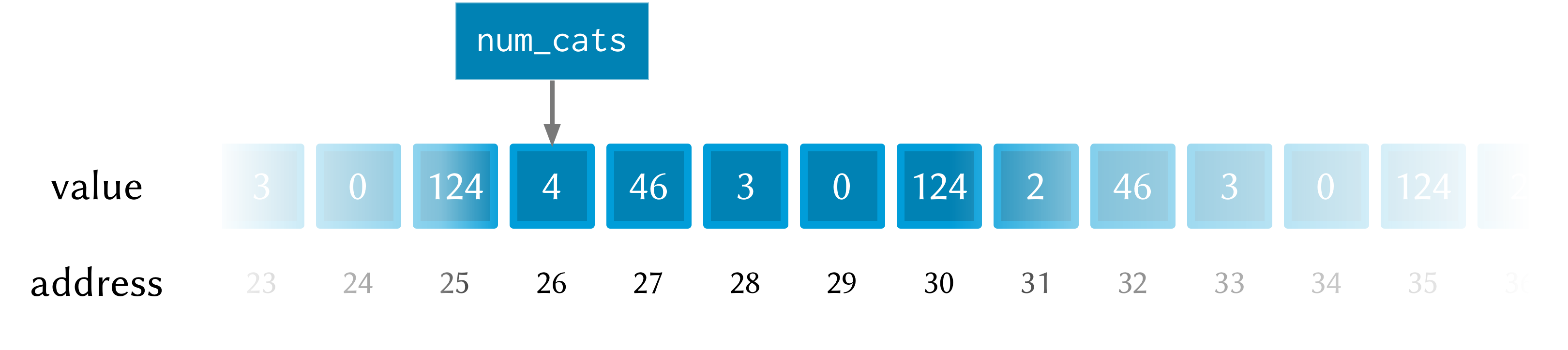In this image the computer’s memory is represented by the blue boxes. Each box has an address (the number below the box), an in this picture you can see that this is only a subset of the total number of memory boxes (in a modern computer there are millions of memory boxes).

The variable `num_cats` keeps track of the value that we’re interested in. In this case the address of that value is ‘memory location 26’, but it could easily be any other location (and indeed will almost certainly be different if the closure `print_num_cats` is called again).

Once a variable exists, we can change its value with `set!`:

``````(bind-func print_num_cats2
(lambda ()
(let ((num_cats:i64 4))
(printf "You have %lld cats... " num_cats)
(set! num_cats 13)
(printf "and now you have %lld cats!\n" num_cats))))

(print_num_cats2)
;; prints "You have 4 cats... and now you have 13 cats!"
``````

The `set!` function changes the value of `num_cats`: it sets a new value into the memory location that `num_cats` refers to. In `print_num_cats2` the value of `num_cats` starts out as `4`, so the first `printf` call prints “You have 4 cats…”. The memory at this point might look like this: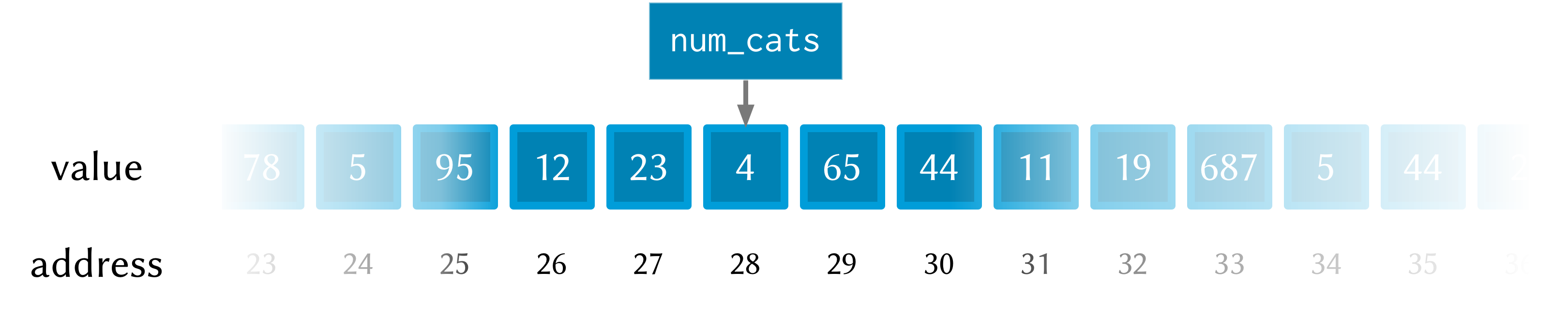But then a new value (`13`) is set into `num_cats` with the call to `set!`, so the second call to `printf` prints “and now you have 13 cats!”. After the call to `set!`, this is what the memory looks like: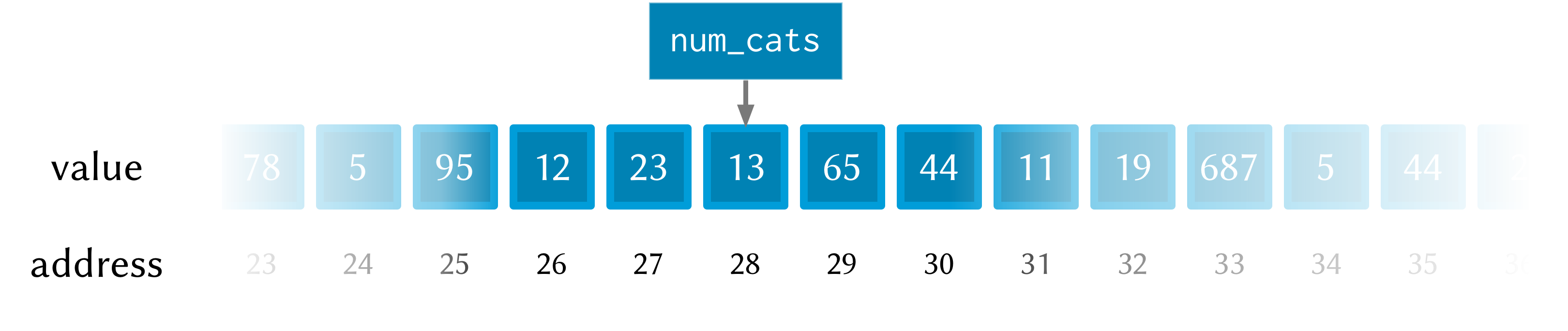Notice how this time the memory address for `num_cats` is different to what it was the previous time (28 rather than 26). This is because the `let` rebinds all its variable-value pairs each time it is entered, and then forgets them when it is exited (that is, when the paren matching the opening paren is reached).

## Pointers: storing memory addresses as values

What we’ve done so far is store the value (how many cats we have) into the variable `num_cats`. The value has an address in memory, but as a programmer we don’t necessarily know what that address is, just that we can refer to the value using the name `num_cats`. It’s important to note that the compiler knows what the address is—in fact as far as the compiler is concerned every variable is just an address. But the compiler allows us to give these variables names, which makes the code much easier to write and understand.

Pointer types in xtlang are indicated with an asterisk (`*`), for example the type `i64*` represents a pointer to a 64-bit integer (sometimes called an `i64`-pointer). With pointers, we actually assign the address itself in a variable. That’s the reason it’s called a pointer: because it points to (is a reference to) the value.

Let’s update our code for printing the number of cats to use a pointer to the value, rather than the value itself. Notice how the type of `num_cats_ptr` is `i64*` (a pointer to an `i64`) rather than just an `i64` like it was before.

``````(bind-func print_num_cats3
(lambda ()
(let ((num_cats_ptr:i64* (zalloc)))
(printf "You have %lld cats!\n" num_cats_ptr))))

(print_num_cats3) ;; prints "You have 4555984976 cats!"
``````

There are a couple of other changes to the code. Firstly, we no longer bind the value straight away (as we were doing with `(num_cats:i64 4)`), but instead we make a call to `zalloc`. This is the way to get pointers in xtlang: through a call to an ‘alloc’ function. `zalloc` is a function which ‘allocates’ and returns the address (i.e. a pointer) of some memory which can be used to store the value in. This address is the assigned to the variable `num_cats_ptr`, just like the number `4` was assigned to `num_cats` in the earlier examples. The orange bar on the variable name indicates that it’s a pointer.

So why does `print_num_cats3` print such a weird (on my machine: 4555984976 cats!) answer? Well, it’s because we’re trying to print it as an `i64` value (using `%lld` in the `printf` format string), but it’s not an `i64` value—it’s the address of a memory location where an `i64` value is located. On a 64-bit system (such as the laptop I’m writing this blog post on) the pointers are actually 64-bit integers, because an integer is a pretty sensible way to store an address.

Incidentally, this is one of the key benefits (and driving forces behind) the switch from 32 to 64 bit architectures—the need for more memory addresses. If a pointer is a 32 bit integer, then you can only ‘address’ about 4.3 billion (232) different memory locations. This might seem like a lot, but as more and more computers came with more than 4.3Gb of RAM installed, so the need for 64-bit pointers became more pressing. There are workarounds, but having a larger addressable space is a key benefit of 64-bit architectures. And it helps to remember that pointers are just integers, but they’re not like the int types that we use to store and manipulate data.

In `print_num_cats3` we don’t set any value into that location, we only deal with the address. In fact, the memory this address points to is referred to as uninitialised, which is a name for memory that has been allocated but hasn’t had any values set into it. In Extempore, uninitialised memory will be ‘zeroed out’, meaning all of the bits will be set to `0`. So for an `i64` this will be the integer value `0`.

After the call to `zalloc`, the memory therefore will look like this (the value is now shown in a different coloured box, to indicate it’s an `i64*` pointer type and not an `i64` value type)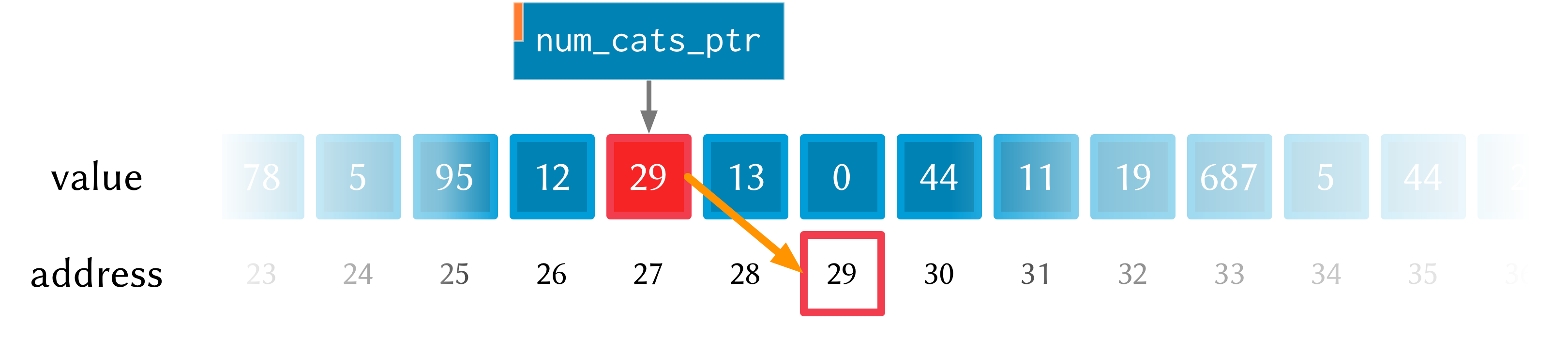This is cool, we can see that the value in memory location 27 is actually the address 29, and the value of 29 is `0` because we haven’t initialised it yet. So, remember how in `print_num_cats2` we used `set!` to set a value into the variable `num_cats`? Well, we can do a similar thing with the pointer `num_cats_ptr` using the function `pset!`. `pset!` takes three arguments: a pointer, an index (which is zero in this next example, but I’ll get to what the index means in the next section) and a value. The value must be of the right type: e.g. if the pointer is a pointer to a double (a `double*`) then the value must be a `double`.

``````(bind-func print_num_cats4
(lambda ()
(let ((num_cats_ptr:i64* (zalloc)))
(pset! num_cats_ptr 0 5)
(printf "You have %lld cats!\n" (pref num_cats_ptr 0)))))

(print_num_cats4) ;; prints "You have 5 cats!"
``````

Great—the function now prints the right number of cats (in this case `5`), so things are working properly again. After the `pset!` call, the memory will look like this (the only difference from last time is that the value 5 is stored in address 29, just as it should be).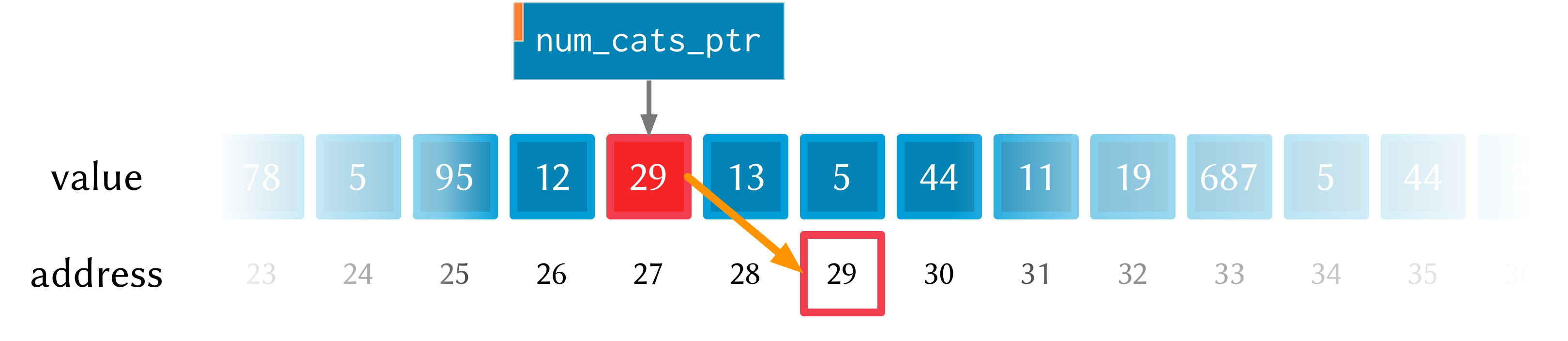Notice also that in `print_num_cats4` we don’t pass `num_cats_ptr` directly to `printf`, we do it through a call to `pref`. Whereas `pset!` is for writing values into memory locations, `pref` is for reading them out. Like `pset!`, pref takes a pointer as the first argument and an offset for the second argument. In this way, we can read and write `i64` values to the memory location without actually having a variable of type `i64` (which we did with `num_cats` in the `print_num_cats` and `print_num_cats2`). All this is possible because we have a pointer variable (`num_cats_ptr`) which gives us a place to load and store the data.

## Buffers and pointer arithmetic

In all the examples so far, we’ve only used a pointer to a single value. This has worked fine, but you might have been wondering why we bothered, because assigning values directly to variables (as we did in the first couple of examples) seemed to work just fine.

One thing that pointers and alloc’ing allows us to do is work with whole regions in memory, in which we can store lots of values. Say we want to be able to determine the mean (average) of 3 numbers. One way to do this is to store each of the different numbers with its own name.

``````(bind-func mean1
(lambda ()
(let ((num1:double 4.5)
(num2:double 3.3)
(num3:double 7.9))
(/ (+ num1 num2 num3)
3.0))))

;; call the function
(mean1) ;; returns 5.233333
``````

The `let` form binds the (`double`) values `4.5`, `3.3` and `7.9` to the names `num1`, `num2` and `num3`. Then, all three values are added together (with `+`) and then divided by `3.0` (with `/`). Now, this code does give the right answer, but it’s easy to see how things would get out of hand if we wanted to find the mean of 5, 20 or one million values. What we really want is a way to give one name to all the values we’re interested in, rather than having to refer to all the values by name individually. And to do that, we can use a pointer.

``````(bind-func mean2
(lambda ()
(let ((num_ptr:double* (zalloc 3)))
;; set the values into memory
(pset! num_ptr 0 4.5)
(pset! num_ptr 1 3.3)
(pset! num_ptr 2 7.9)
;; together, and then divide  by 3
(/ (+ (pref num_ptr 0)
(pref num_ptr 1)
(pref num_ptr 2))
3.0))))

(mean2) ;; returns 5.233333
``````

In `mean2`, we pass an integer argument (in this case `3`) to `zalloc`. `zalloc` then allocates enough memory to fit 3 `double` values. The pointer that gets returned is still only a pointer to the first of these memory slots. And this is where the second ‘offset’ argument to `pref` and `pset!` come in.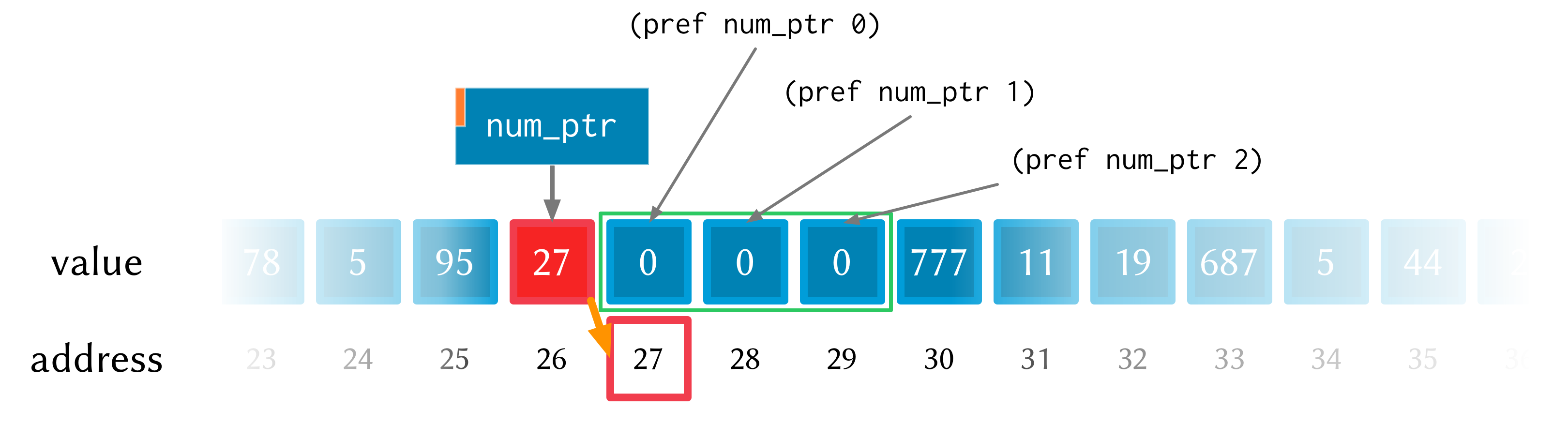See how the repeated calls to `pset!` and `pref` above have different offset values? Well, that’s because the offset argument allows you to get and set values ‘further into’ the memory returned by `(zalloc 3)`. This isn’t anything magical, they just add the offset to the memory address.

There is a helpful function called `pfill!` for filling multiple values into memory (multiple calls to `pset!`) as we did in the above example. Rewriting `mean2` to use `pfill!`:

``````(bind-func mean3
(lambda ()
(let ((num_ptr:double* (zalloc 3)))
;; set the values into memory
(pfill! num_ptr 4.5 3.3 7.9)
;; together, and then divide  by 3
(/ (+ (pref num_ptr 0)
(pref num_ptr 1)
(pref num_ptr 2))
3.0))))

(mean3) ;; returns 5.233333
``````

Finally, one more useful way to fill values into a chunk of memory is using a `dotimes` loop. To do this, we need to bind a helper value `i` to use as an index for the loop. This function allocates enough memory for 5 `i64` values, and just fills it with ascending numbers:

``````(bind-func ptr_loop
(lambda ()
(let ((num_ptr:i64* (zalloc 5))
(i:i64 0))
;; loop from i = 0 to i = 4
(dotimes (i 5)
(pset! num_ptr i i))
(pref num_ptr 3))))

(ptr_loop) ;; returns 3
``````

After the `dotimes` the memory will look like this: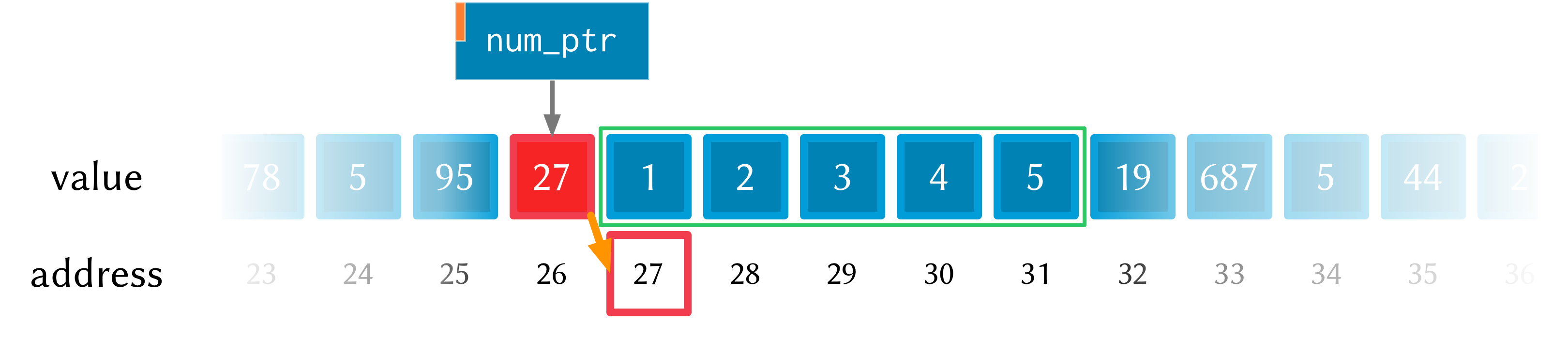There’s one more useful function for working with pointers: `pref-ptr`. Where `(pref num_ptr 3)` returns the value of the 4th element of the chunk of memory pointed to by `num_ptr`, `(pref-ptr num_ptr 3)` returns the address of that value (a pointer to that value). So, in the example above, `num_ptr` points to memory address 27, so `(pref num_ptr 2)` would point to memory address 29. `(pref (pref-ptr num_ptr n) 0)` is the same as `(pref (pref-ptr num_ptr 0) n)` for any integer n.

## Pointers to higher-order types

The xtlang type system <types> has both primitive types (floats and ints) and higher-order types like tuples, arrays and closures. Higher-order in this instance just means that they are made up of other types, although these component types may be themselves higher-order types.

As an example of an aggregate type, consider a 2 element tuple. Tuples are (fixed-length) n-element structures, and are declared with angle brackes (`<>`). So a tuple with an `i64` as the first element and a double as the second element would have the type signature `<i64,double>`. Getting and setting tuple elements is done with `tref` and `tset!` respectively, which both work exactly like `pref=/=pset!` except the first argument has to be a pointer to a tuple.

``````(bind-func print_tuples
(lambda ()
;; step 1: allocate memory for 2 tuples
(let ((tup_ptr:<i64,double>* (zalloc 2)))
;; step 2: initialise tuples
(tset! (pref-ptr tup_ptr 0) 0 2)         ; tuple 1, element 1
(tset! (pref-ptr tup_ptr 0) 1 2.0)       ; tuple 1, element 2
(tset! (pref-ptr tup_ptr 1) 0 6)         ; tuple 2, element 1
(tset! (pref-ptr tup_ptr 1) 1 6.0)       ; tuple 2, element 2
;; step 3: read & print tuple values
(printf "tup_ptr = <%lld,%f>\n"
(tref (pref-ptr tup_ptr 0) 0)    ; tuple 1, element 1
(tref (pref-ptr tup_ptr 0) 1))   ; tuple 1, element 2
(printf "tup_ptr = <%lld,%f>\n"
(tref (pref-ptr tup_ptr 1) 0)    ; tuple 2, element 1
(tref (pref-ptr tup_ptr 1) 1))))); tuple 2, element 2

(print_tuples) ;; prints
;; tup_ptr = <2,2.000000>
;; tup_ptr = <6,6.000000>
``````

This `print_tuples` example works in 3 basic steps:

1. Allocate memory for two (uninitialised) `<i64,double>` tuples, bind pointer to this memory to `tup_ptr`.
2. Initialise tuples with values (in this case `2` and `2.0` for the first tuple and `6` and `6.0` for the second one). Notice the nested `tset!` and `pref-ptr` calls: `pref-ptr` returns a pointer to the tuple at offset 0 (for the first) and 1 (for the second). This pointer is then passed as the first argument to `tset!`, which fills it with a value at the appropriate element.
3. Read (& print) values back out of the tuples. These should be the values we just set in step 2—and they are.

Let’s have a look at what the memory will look like during the execution of `print_tuples`. After the call to `(zalloc)` (step 1), we have a pointer to a chunk of memory, but the tuples in this memory are uninitialised (indicated by u).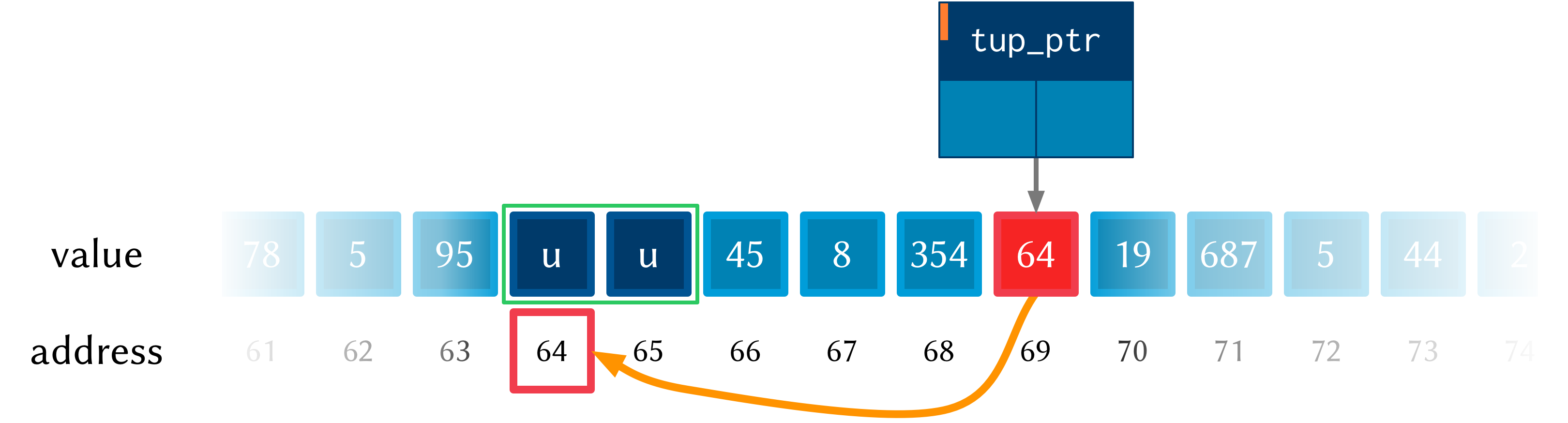After using `pref` and `tset!` in step 2, the values get set into the tuples. Step 3 simply reads these values back out—it doesn’t change the memory.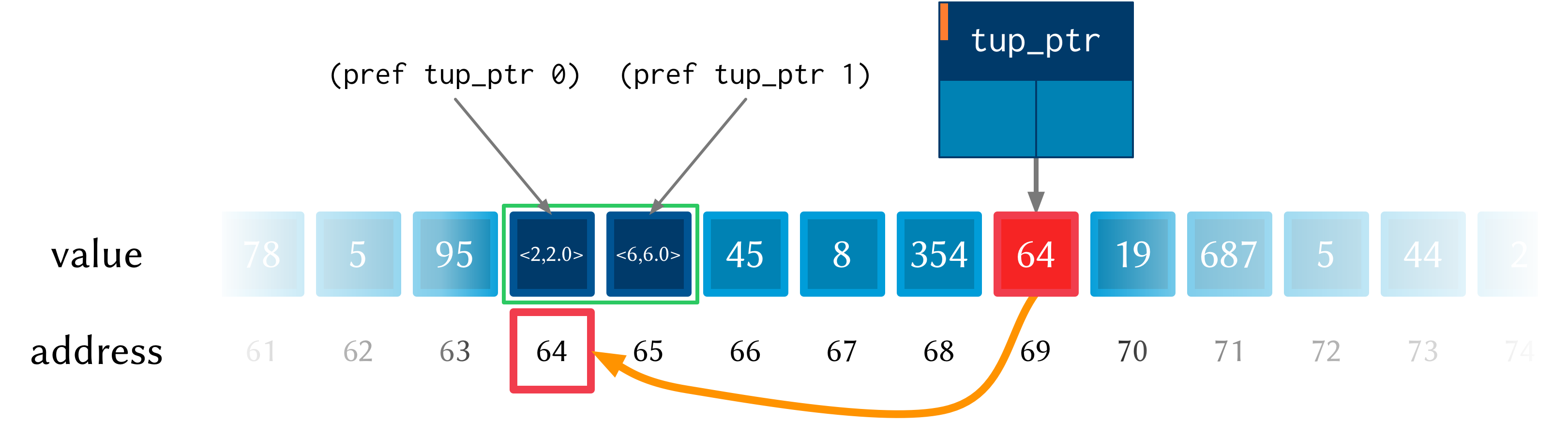• We used `pref_ptr` rather than `pref` in both step 2 and step 3. That’s because `tset!` and `tref` need a pointer to a tuple as their first argument, and if we had used regular `pref` we would have got the tuple itself. This means that we could have just used `tup_ptr` directly instead of `(pref-ptr tup_ptr 0)` in a couple of places, because these two pointers will always be equal (have a think about why this is true).
• There are a few bits of repeated code, for example `(pref-ptr tup_ptr 1)` gets called 4 times. We could have stored this pointer in a temporary variable to prevent these multiple dereferences, how could we have done that (hint: create the new ‘tmp’ pointer in the `let`—make sure it’s of the right type).

There’s one final thing worth saying about pointers in xtlang. Why do pointers even have types? Isn’t the address the same whether it’s an int, a float, a tuple, or some complex custom type stored at that memory address? The reason is to do with something all this talk of memory locations as ‘boxes’ has glossed over: that different types require different amounts of memory to store.

A more accurate (though still simplified) picture of the computer’s memory is to think of the boxes as 8-bit bytes. One bit (a binary digit) is just a `0` or a `1`, and a byte is made up of 8 bits, for example `11001011`. These are just base-2 numerals, so `5` in decimal is `101`, and although they are difficult for humans to read (unless you’re used to them), computers live and breathe binary digits.

This is why the integer types all have numbers associated with them—the number represents the number of bytes used to store the integer. So `i64` requires 64 bits, while an `i8` only requires 8. The reason for having different sizes is that larger sizes take up more room (more bytes) in memory, but can also store larger values (n bits can store 2n different numbers). All the other types have sizes, too: a `float` is 32 bits for instance, and the number of bits required to represent an aggregate type like a tuple or an array is (at least) the sum of the sizes of their components.

So, reconsidering our very first example, where we stored an `i64` value of `4` to represent how many cats we had, a more accurate diagram of the actual memory layout in this situation is: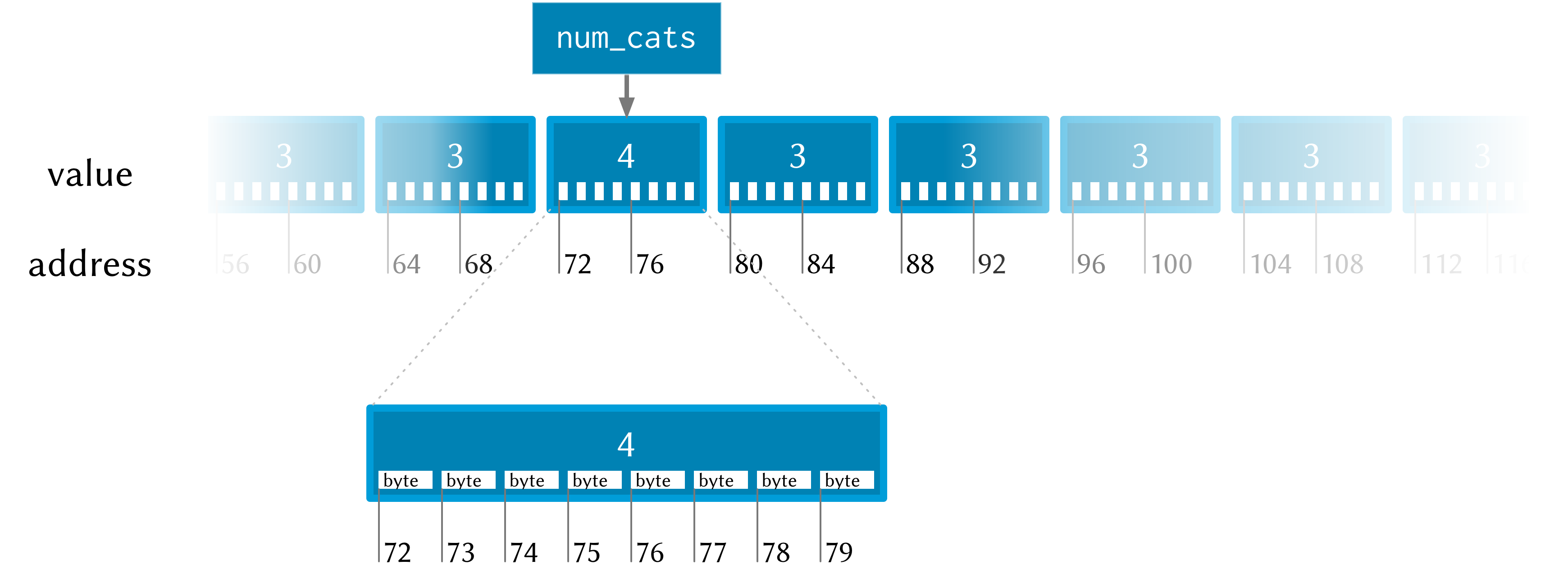See how each `i64` value takes up 8 bytes? Also, each byte has a memory addresses, so the start of each `i64` in memory is actually 8 bytes along from the previous one.

Now, consider the layout of an aggregate type like a tuple: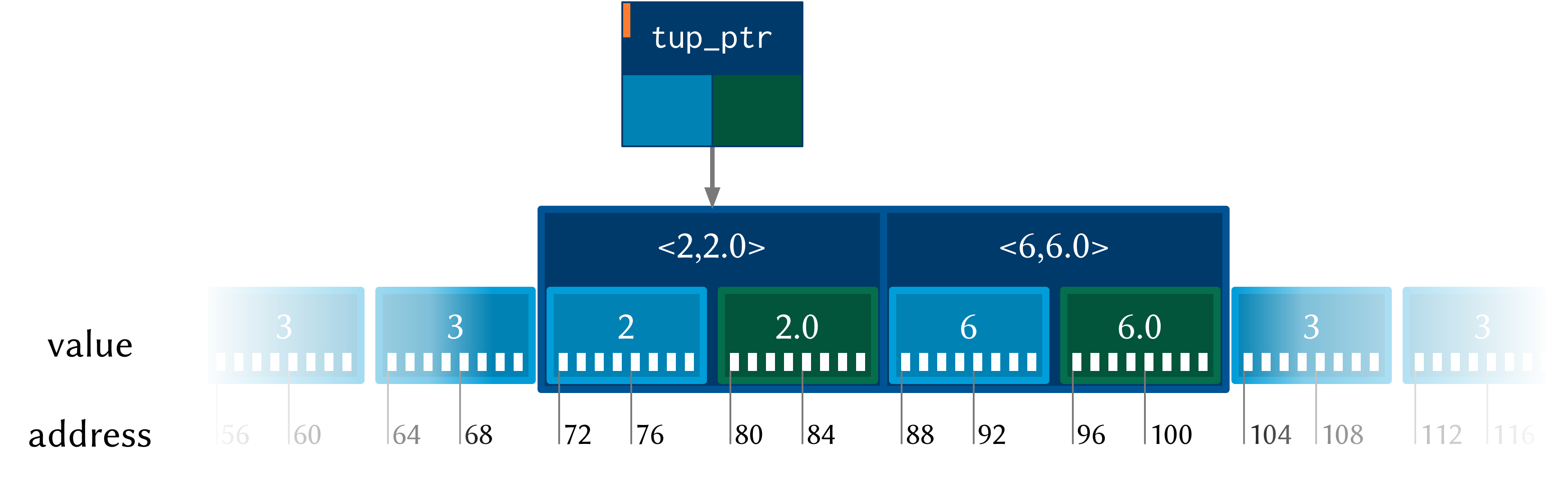Each tuple contains (and therefore takes up the space of) an `i64` and a `double`. So the actual memory address offset between the beginning of consecutive tuples is 16 bytes. But `pref` still works the same as in the `i64*` case. `(pref tup_ptr 1)` gets the second tuple—it doesn’t try and read a tuple from ‘half way in’.

This is one reason why pointers have types: the type of the pointer tells `pref` how far to jump to get between consecutive elements (this value is called the stride). This becomes increasingly helpful when working with pointers to compound types: no-one wants figure out (and keep track of) the size of a tuple like `<i32,i8,|17,double|*,double>` and calculate the stride manually.

## Other benefits of using pointers

There are a few other situations where being able to pass pointers around is really handy.

• When the chunks of memory we’re dealing with are large, copying them around in memory becomes expensive (in the ‘time taken’ sense). So, if lots of different functions need to work on the same data, instead of copying it around so that each function has its own copy of the data, they can just pass around pointers to the same chunk of data. This means that each function needs to be a good citizen and not stuff up things for the others, but if you’re careful this can be a huge performance benefit.
• You can programatically determine the amount of memory to allocate, which is something you can’t to with xtlang’s array types.